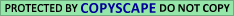## A.6. The Randomizations

The 999,999 random permutations of the 32 personalities were chosen in accordance with Algorithm P of Knuth, page 125. The pseudorandom generator required as input to this algorithm was that provided by Turbo-Pascal 5.0 of Borland Inter Inc. This, in turn, requires a seed consisting of 32 binary bits; that is, an integer with 32 digits when written to the base 2. To generate this seed, each of three prominent scientists was asked to provide such an integer, just before the calculation was carried out. The first of the three tossed a coin 32 times; the other two used the parities of the digits in widely separated blocks in the decimal expansion of p. The three resulting integers were added modulo 232. The resulting seed was 01001 10000 10011 11100 00101 00111 11. (KNUTH, D. E. (1969). The Art of Computer Programming 2. Addison-Wesley, Reading, MA.)

The control text R was constructed by permuting the 78,064 letters of G with a single random permutation, generated as in the previous paragraph. In this case, the seed was picked arbitrarily to be the decimal integer 10 (i.e., the binary integer 1010). The control text W was constructed by permuting the words of G in exactly the same way and with the same seed, while leaving the letters within each word unpermuted. The control text V was constructed by permuting the verses of G in the same way and with the same seed, while leaving the letters within each verse unpermuted.

The control text U was constructed by permuting the words within each verse of G in the same way and with the same seed, while leaving unpermuted the letters within each word, as well as the verses. More precisely, the Algorithm P of Knuth that we used requires n - 1 random numbers to produce a random permutation of n items. The pseudorandom generator of Borland that we used produces, for each seed, a long string of random numbers. Using the binary seed 1010, we produced such a long string. The first six numbers in this string were used to produce a random permutation of the seven words constituting the first verse of Genesis. The next 13 numbers (i.e., the 7th through the 19th random numbers in the string produced by Borland) were used to produce a random permutation of the 14 words constituting the second verse of Genesis, and so on. (KNUTH, D. E. (1969). The Art of Computer Programming 2. Addison-Wesley, Reading, MA.)

Click Here to Go To Table 1 Page.